Area as a sum

Chapter 7 Class 12 Integrals
Serial order wise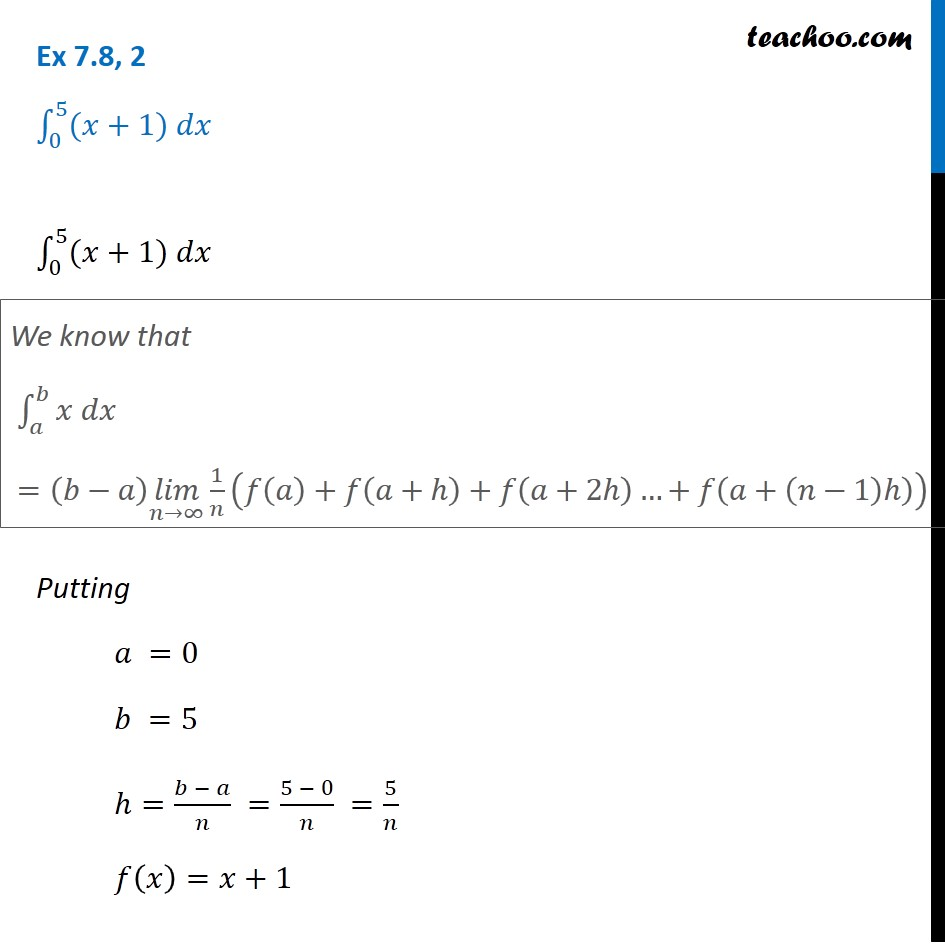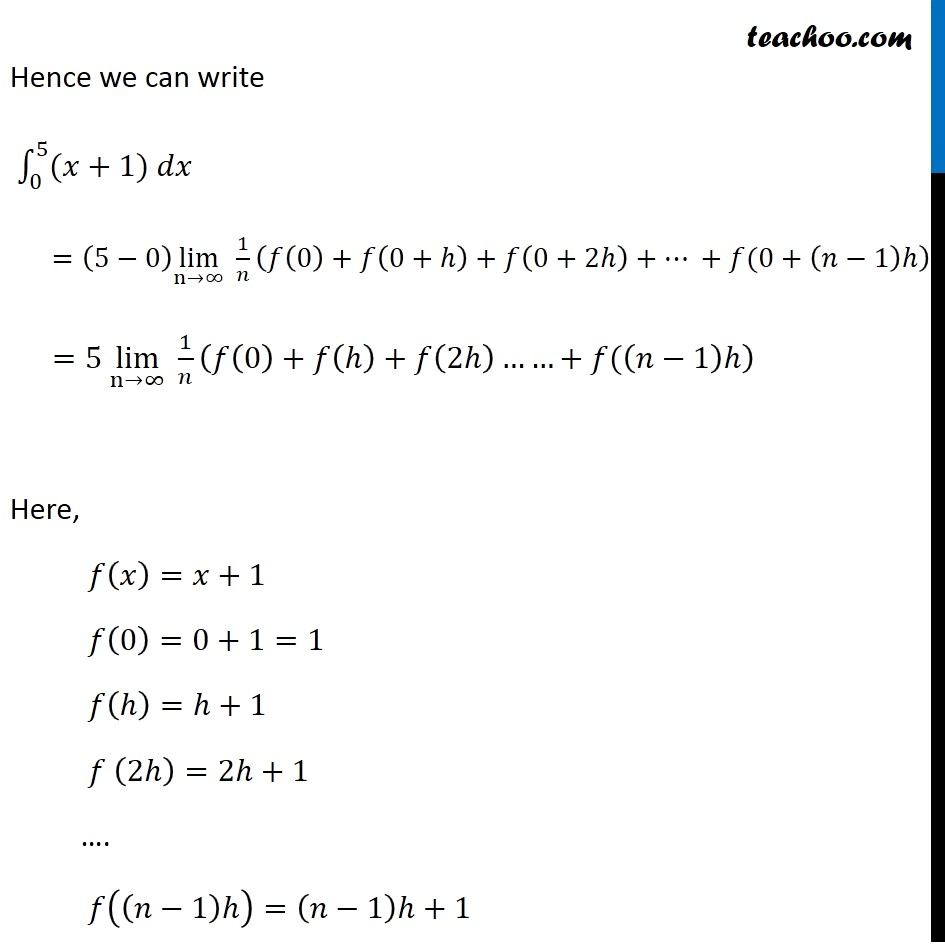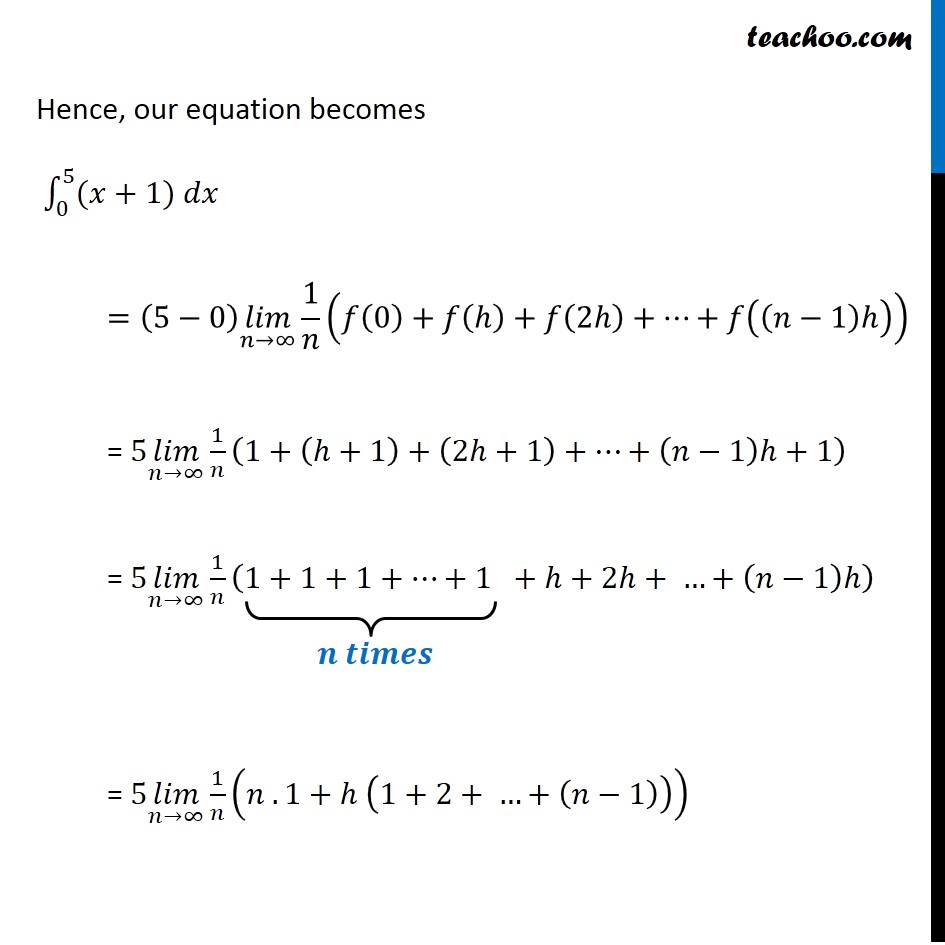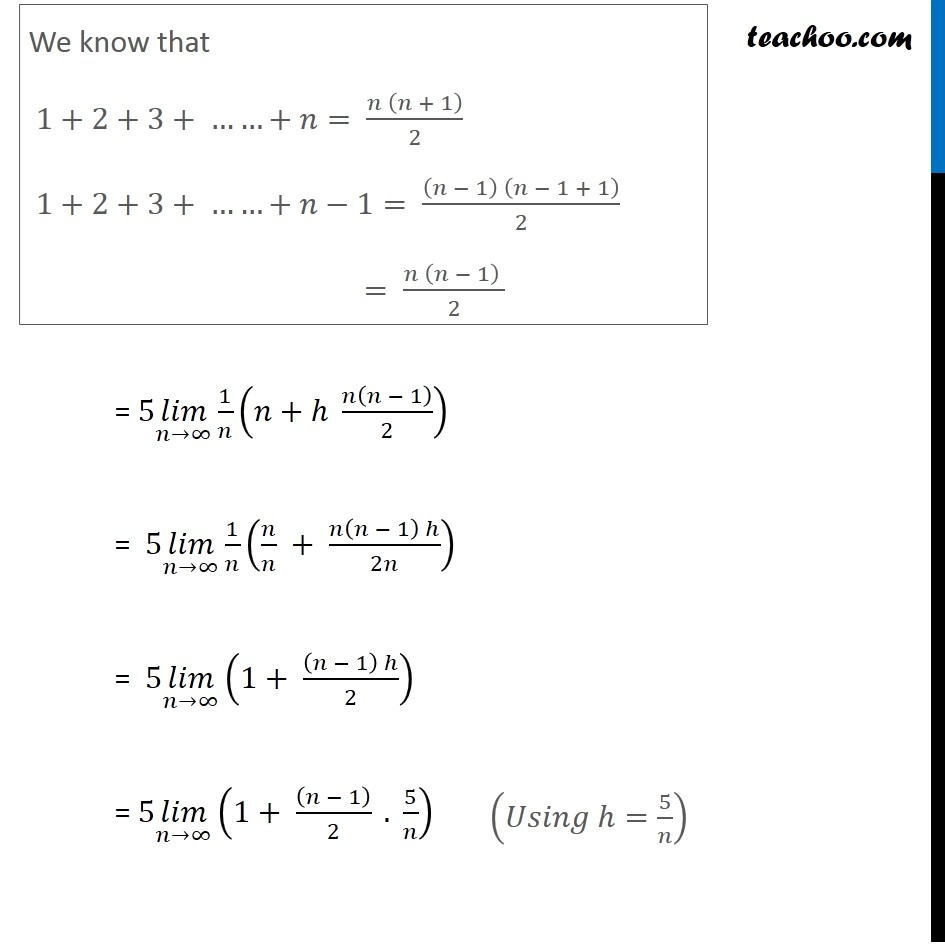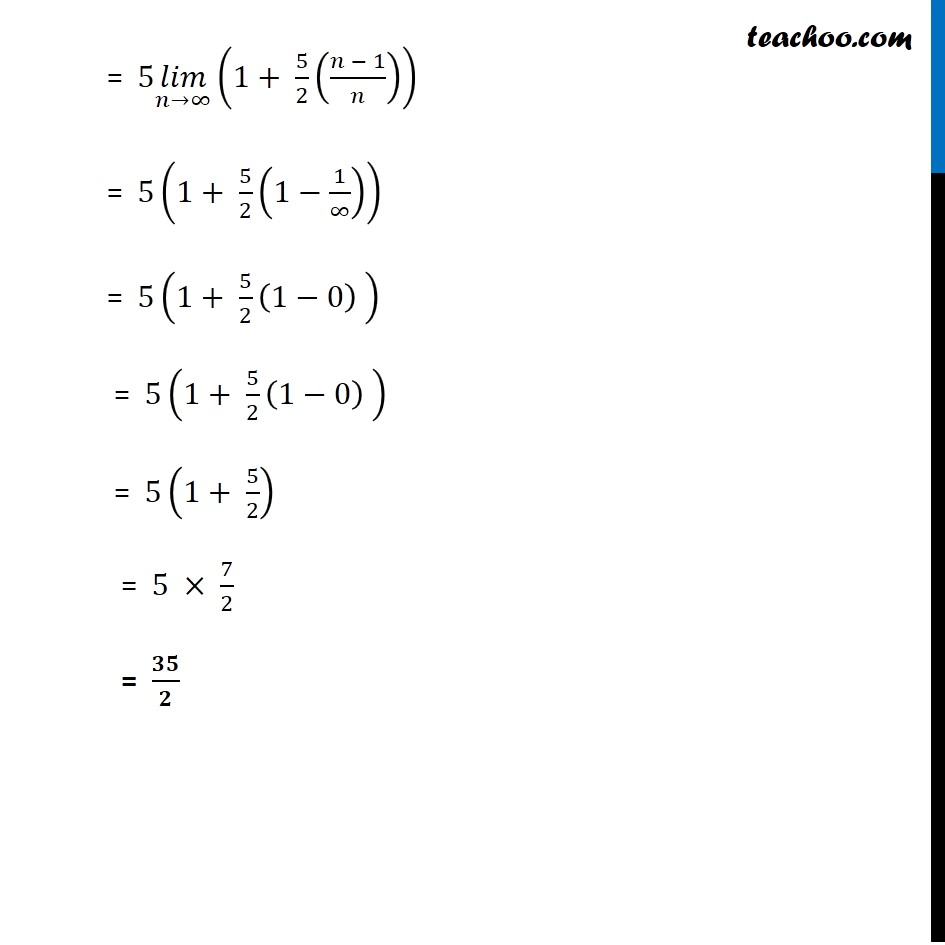Learn in your speed, with individual attention - Teachoo Maths 1-on-1 Class

### Transcript

Question 2 ∫1_0^5▒〖(𝑥+1) 𝑑𝑥〗 ∫1_0^5▒〖(𝑥+1) 𝑑𝑥〗 Putting 𝑎 =0 𝑏 =5 ℎ=(𝑏 − 𝑎)/𝑛 =(5 − 0)/𝑛 =5/𝑛 𝑓(𝑥)=𝑥+1 We know that ∫1_𝑎^𝑏▒〖𝑥 𝑑𝑥〗 =(𝑏−𝑎) (𝑙𝑖𝑚)┬(𝑛→∞) 1/𝑛 (𝑓(𝑎)+𝑓(𝑎+ℎ)+𝑓(𝑎+2ℎ)…+𝑓(𝑎+(𝑛−1)ℎ)) Hence we can write ∫1_0^5▒〖(𝑥+1) 𝑑𝑥〗 =(5−0) lim┬(n→∞) 1/𝑛 (𝑓(0)+𝑓(0+ℎ)+𝑓(0+2ℎ)+… +𝑓(0+(𝑛−1)ℎ) =5 lim┬(n→∞) 1/𝑛 (𝑓(0)+𝑓(ℎ)+𝑓(2ℎ)……+𝑓((𝑛−1)ℎ) Here, 𝑓(𝑥)=𝑥+1 𝑓(0)=0+1=1 𝑓(ℎ)=ℎ+1 𝑓 (2ℎ)=2ℎ+1 …. 𝑓((𝑛−1)ℎ)=(𝑛−1)ℎ+1 Hence, our equation becomes ∫1_0^5▒〖(𝑥+1) 𝑑𝑥〗 =(5−0) (𝑙𝑖𝑚)┬(𝑛→∞) 1/𝑛 (𝑓(0)+𝑓(ℎ)+𝑓(2ℎ)+…+𝑓((𝑛−1)ℎ)) = 5 (𝑙𝑖𝑚)┬(𝑛→∞) 1/𝑛 (1+(ℎ+1)+(2ℎ+1)+…+(𝑛−1)ℎ+1) = 5 (𝑙𝑖𝑚)┬(𝑛→∞) 1/𝑛 (1+1+1+…+1 +ℎ+2ℎ+ …+(𝑛−1)ℎ) = 5 (𝑙𝑖𝑚)┬(𝑛→∞) 1/𝑛 (𝑛 . 1+ℎ (1+2+ …+(𝑛−1))) We know that 1+2+3+ ……+𝑛= (𝑛 (𝑛 + 1))/2 1+2+3+ ……+𝑛−1= ((𝑛 − 1) (𝑛 − 1 + 1))/2 = (𝑛 (𝑛 − 1) )/2 = 5 (𝑙𝑖𝑚)┬(𝑛→∞) 1/𝑛 (𝑛+ℎ 𝑛(𝑛 − 1)/2) = 5 (𝑙𝑖𝑚)┬(𝑛→∞) 1/𝑛 (𝑛/𝑛 + (𝑛(𝑛 − 1) ℎ)/2𝑛) = 5 (𝑙𝑖𝑚)┬(𝑛→∞) (1+ ((𝑛 − 1) ℎ)/2) = 5 (𝑙𝑖𝑚)┬(𝑛→∞) (1+ ((𝑛 − 1))/2 . 5/𝑛) (𝑈𝑠𝑖𝑛𝑔 ℎ=5/𝑛) = 5 (𝑙𝑖𝑚)┬(𝑛→∞) (1+ 5/2 ((𝑛 − 1)/𝑛)) = 5(1+ 5/2 (1−1/∞)) = 5(1+ 5/2 (1−0) ) = 5(1+ 5/2 (1−0) ) = 5(1+ 5/2) = 5 × 7/2 = 𝟑𝟓/𝟐